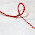## Tuesday, August 9, 2011

### Calculate GSM (w/o machine)

Learn Apparel Merchandising, Learn Fashion design.
Calculate GSM from the data given

Total Weight of fabric                 = 15.5 Kgs
Length of fabric                          = 35 meters
Width of fabric in open form      = 65 inches

Solution:
First we will calculate area of the fabric

Fabric length      = 35 meters
Fabric width      = 65 inches or 1.65 meters
Fabric area        = Length * width
=35 * 1.65
=57.75 meters square

Weight of 57.75  Meter square is 15.5 kgs or 15500 grams
So weight of one square meter

= 15500/57.75
= 268.39 grams per meter square of GSM of the fabric

Thanks

1.2.